• 最小二乘法Least Square Method，做为分类回归算法的基础，有着悠久的历史（由马里·勒让德于1806年提出）。它通过最小化误差的平方和寻找数据的最佳函数匹配。利用最小二乘法可以简便地求得未知的数据，并使得这些...
• 使用Python编程，分别根据梯度法和最小二乘法求解多元函数问题分别使用梯度下降法和最小二乘法求解多元函数并进行比较，这里使用jupyter notebook平台进行Python编程一、题目描述二、使用梯度下降法求解多元函数（一...
使用Python编程，分别根据梯度法和最小二乘法求解多元函数问题分别使用梯度下降法和最小二乘法求解多元函数并进行比较，这里使用jupyter notebook平台进行Python编程一、题目描述二、使用梯度下降法求解多元函数（一）梯度下降法基本原理（二）梯度下降法公式（三）Python代码实现1、导入要使用到的库、定义变量并赋值2、代价函数3、使用梯度下降法求解多元函数系数4、打印系数和方程5、绘制线性回归方程拟合图6、完整代码7、运行结果三、使用最小二乘法求解多元函数（一）最小二乘法基本原理（二）Python代码实现1、自行推导过程编写代码运行结果2、采用sklearn库运行结果（四）将梯度下降法与最小二乘法进行对比
分别使用梯度下降法和最小二乘法求解多元函数并进行比较，这里使用jupyter notebook平台进行Python编程
一、题目描述
为求得某个地区的商品店的月营业额与店铺的面积、该店距离车站距离的相关性大小，以店铺面积、距离车站的距离、以及月营业额建立线性回归方程，并求解方程，得到相关系数。将表中的数据录入到Excel中，编程时会用到这些数据，如下：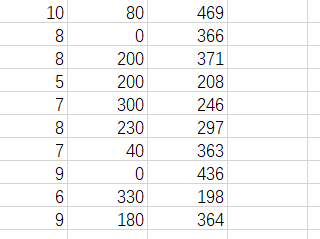二、使用梯度下降法求解多元函数
（一）梯度下降法基本原理
梯度下降法又称最速下降法，是求解无约束最优化问题的一种最常用的方法，在对损失函数最小化时经常使用。梯度下降法是一种迭代算法。选取适当的初值x(0)，不断迭代，更新x的值，进行目标函数的极小化，直到收敛。由于负梯度方向时使函数值下降最快的方向，在迭代的每一步，以负梯度方向更新x的值，从而达到减少函数值的目的。
（二）梯度下降法公式（三）Python代码实现
1、导入要使用到的库、定义变量并赋值
import numpy as np
from matplotlib import pyplot as plt
from mpl_toolkits.mplot3d import Axes3D
data=np.genfromtxt('D:/area_distance.csv',delimiter=',')
x_data=data[:,:-1]
y_data=data[:,2]
#定义学习率、斜率、截据
#设方程为y=theta1x1+theta2x2+theta0
lr=0.00001
theta0=0
theta1=0
theta2=0
#定义最大迭代次数，因为梯度下降法是在不断迭代更新k与b
epochs=10000

2、代价函数
def compute_error(theta0,theta1,theta2,x_data,y_data):
totalerror=0
for i in range(0,len(x_data)):#定义一共有多少样本点
totalerror=totalerror+(y_data[i]-(theta1*x_data[i,0]+theta2*x_data[i,1]+theta0))**2
return totalerror/float(len(x_data))/2

3、使用梯度下降法求解多元函数系数
def gradient_descent_runner(x_data,y_data,theta0,theta1,theta2,lr,epochs):
m=len(x_data)
for i in range(epochs):
theta0_grad=0
theta1_grad=0
theta2_grad=0
for j in range(0,m):
theta0_grad-=(1/m)*(-(theta1*x_data[j,0]+theta2*x_data[j,1]+theta2)+y_data[j])
theta1_grad-=(1/m)*x_data[j,0]*(-(theta1*x_data[j,0]+theta2*x_data[j,1]+theta0)+y_data[j])
theta2_grad-=(1/m)*x_data[j,1]*(-(theta1*x_data[j,0]+theta2*x_data[j,1]+theta0)+y_data[j])
theta0=theta0-lr*theta0_grad
theta1=theta1-lr*theta1_grad
theta2=theta2-lr*theta2_grad
return theta0,theta1,theta2

4、打印系数和方程
theta0,theta1,theta2=gradient_descent_runner(x_data,y_data,theta0,theta1,theta2,lr,epochs)
print('迭代次数：{0} 学习率：{1}之后 a0={2},a1={3},a2={4},代价函数为{5}'.format(epochs,lr,theta0,theta1,theta2,compute_error(theta0,theta1,theta2,x_data,y_data)))
print("多元线性回归方程为:y=",theta1,"X1+",theta2,"X2+",theta0)

5、绘制线性回归方程拟合图
#画图
ax=plt.figure().add_subplot(111,projection='3d')
ax.scatter(x_data[:,0],x_data[:,1],y_data,c='r',marker='o')
x0=x_data[:,0]
x1=x_data[:,1]
#生成网格矩阵
x0,x1=np.meshgrid(x0,x1)
z=theta0+theta1*x0+theta2*x1
#画3d图
ax.plot_surface(x0,x1,z)
ax.set_xlabel('area')
ax.set_ylabel('distance')
ax.set_zlabel("Monthly turnover")
plt.show()

6、完整代码
import numpy as np
from matplotlib import pyplot as plt
from mpl_toolkits.mplot3d import Axes3D
data=np.genfromtxt('D:/area_distance.csv',delimiter=',')
x_data=data[:,:-1]
y_data=data[:,2]
#定义学习率、斜率、截据
#设方程为y=theta1x1+theta2x2+theta0
lr=0.00001
theta0=0
theta1=0
theta2=0
#定义最大迭代次数，因为梯度下降法是在不断迭代更新k与b
epochs=10000
#定义最小二乘法函数-损失函数（代价函数）
def compute_error(theta0,theta1,theta2,x_data,y_data):
totalerror=0
for i in range(0,len(x_data)):#定义一共有多少样本点
totalerror=totalerror+(y_data[i]-(theta1*x_data[i,0]+theta2*x_data[i,1]+theta0))**2
return totalerror/float(len(x_data))/2
#梯度下降算法求解参数
def gradient_descent_runner(x_data,y_data,theta0,theta1,theta2,lr,epochs):
m=len(x_data)
for i in range(epochs):
theta0_grad=0
theta1_grad=0
theta2_grad=0
for j in range(0,m):
theta0_grad-=(1/m)*(-(theta1*x_data[j,0]+theta2*x_data[j,1]+theta2)+y_data[j])
theta1_grad-=(1/m)*x_data[j,0]*(-(theta1*x_data[j,0]+theta2*x_data[j,1]+theta0)+y_data[j])
theta2_grad-=(1/m)*x_data[j,1]*(-(theta1*x_data[j,0]+theta2*x_data[j,1]+theta0)+y_data[j])
theta0=theta0-lr*theta0_grad
theta1=theta1-lr*theta1_grad
theta2=theta2-lr*theta2_grad
return theta0,theta1,theta2
#进行迭代求解
theta0,theta1,theta2=gradient_descent_runner(x_data,y_data,theta0,theta1,theta2,lr,epochs)
print('迭代次数：{0} 学习率：{1}之后 a0={2},a1={3},a2={4},代价函数为{5}'.format(epochs,lr,theta0,theta1,theta2,compute_error(theta0,theta1,theta2,x_data,y_data)))
print("多元线性回归方程为:y=",theta1,"X1+",theta2,"X2+",theta0)
#画图
ax=plt.figure().add_subplot(111,projection='3d')
ax.scatter(x_data[:,0],x_data[:,1],y_data,c='r',marker='o')
x0=x_data[:,0]
x1=x_data[:,1]
#生成网格矩阵
x0,x1=np.meshgrid(x0,x1)
z=theta0+theta1*x0+theta2*x1
#画3d图
ax.plot_surface(x0,x1,z)
ax.set_xlabel('area')
ax.set_ylabel('distance')
ax.set_zlabel("Monthly turnover")
plt.show()

7、运行结果三、使用最小二乘法求解多元函数
（一）最小二乘法基本原理
在我们研究两个变量（x,y）之间的相互关系时，通常可以得到一系列成对的数据（x1,y1.x2,y2… xm,ym）；将这些数据描绘在x -y直角坐标系中，若发现这些点在一条直线附近，可以令这条直线方程为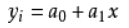（式1-1）
其中：a0、a1 是任意实数
为建立这直线方程就要确定a0和a1，应用《最小二乘法原理》，将实测值Yi与利用计算值Yj（Yj=a0+a1Xi）（式1-1）的离差（Yi-Yj）的平方和 最小为“优化判据”。
令：φ = （式1-2)
把（式1-1）代入（式1-2）中得：
φ = （式1-3)
当 最小时，可用函数 φ 对a0、a1求偏导数，令这两个偏导数等于零。
∑2(a0 + a1Xi - Yi）=0（式1-4)
∑2Xi（a0 +a1Xi - Yi）=0（式1-5)
亦即：
na0 + （∑Xi ) a1 = ∑Yi （式1-6)
（∑Xi ) a0 + （∑Xi^2 ) a1 = ∑（Xi*Yi) （式1-7)
得到的两个关于a0、 a1为未知数的两个方程组，解这两个方程组得出：
a0 = （∑Yi) / n - a1（∑Xi) / n （式1-8)
a1 = [n∑(Xi Yi) - （∑Xi ∑Yi)] / (n∑Xi^2 -∑Xi∑Xi)（式1-9)
这时把a0、a1代入（式1-1）中， 此时的(式1-1）就是我们回归的一元线性方程即：数学模型。
在回归过程中，回归的关联式不可能全部通过每个回归数据点（x1,y1. x2,y2…xm,ym），为了判断关联式的好坏，可借助相关系数“R”，统计量“F”，剩余标准偏差“S”进行判断；“R”越趋近于 1 越好；“F”的绝对值越大越好；“S”越趋近于 0 越好。
R = [∑XiYi - m （∑Xi / m）（∑Yi / m)]/ SQR{[∑Xi2 - m （∑Xi / m)2][∑Yi2 - m （∑Yi / m)2]} （式1-10) *
在（式1-10）中，m为样本容量，即实验次数；Xi、Yi分别为任意一组实验数据X、Y的数值。
（二）Python代码实现
1、自行推导过程编写代码
import numpy as np
import matplotlib.pyplot as plt
import pandas as pd
import seaborn as sns

%matplotlib inline
data = np.genfromtxt("D:/area_distance.csv",delimiter=",")
X1=data[0:10,0]#面积
X2=data[0:10,1]#离车站的距离
Y=data[0:10,2]#月营业额
#将因变量赋值给矩阵Y1
Y1=np.array([Y]).T
#为自变量系数矩阵X赋值
X11=np.array([X1]).T
X22=np.array([X2]).T
A=np.array([,,,,,,,,,])#创建系数矩阵
B=np.hstack((A,X11))#将矩阵a与矩阵X11合并为矩阵b
X=np.hstack((B,X22))#将矩阵b与矩阵X22合并为矩阵X
#求矩阵X的转置矩阵
X_=X.T
#求矩阵X与他的转置矩阵的X_的乘积
X_X=np.dot(X_,X)
#求矩阵X与他的转置矩阵的X_的乘积的逆矩阵
X_X_=np.linalg.inv(X_X)
#求解系数矩阵W，分别对应截距b、a1、和a2
W=np.dot(np.dot((X_X_),(X_)),Y1)
b=W
a1=W
a2=W
print("系数a1={:.1f}".format(a1))
print("系数a2={:.1f}".format(a2))
print("截距为={:.1f}".format(b))
print("多元线性回归方程为:y={:.1f}".format(a1),"X1+ {:.1f}".format(a2),"X2+{:.1f}".format(b))
#画出线性回归分析图
data1=pd.read_excel('D:\面积距离车站数据.xlsx')
sns.pairplot(data1, x_vars=['area','distance'], y_vars='Y', height=3, aspect=0.8, kind='reg')
plt.show()
#求月销售量Y的和以及平均值y1
sumy=0#因变量的和
y1=0#因变量的平均值
for i in range(0,len(Y)):
sumy=sumy+Y[i]
y1=sumy/len(Y)
#求月销售额y-他的平均值的和
y_y1=0#y-y1的值的和
for i in range(0,len(Y)):
y_y1=y_y1+(Y[i]-y1)
print("营业额-营业额平均值的和为:{:.1f}".format(y_y1))
#求预测值sales1
sales1=[]
for i in range(0,len(Y)):
sales1.append(a1*X1[i]+a2*X2[i]+b)
#求预测值的平均值y2
y2=0
sumy2=0
for i in range(len(sales1)):
sumy2=sumy2+sales1[i]
y2=sumy2/len(sales1)
#求预测值-平均值的和y11_y2
y11_y2=0
for i in range(0,len(sales1)):
y11_y2=y11_y2+(sales1[i]-y2)
print("预测营业额-预测营业额平均值的和为:{:.1f}".format(y11_y2))
#求月销售额y-他的平均值的平方和
Syy=0#y-y1的值的平方和
for i in range(0,len(Y)):
Syy=Syy+((Y[i]-y1)*(Y[i]-y1))
print("Syy={:.1f}".format(Syy))
#求y1-y1平均的平方和
Sy1y1=0
for i in range(0,len(sales1)):
Sy1y1=Sy1y1+((sales1[i]-y2)*(sales1[i]-y2))
print("Sy1y1={:.1f}".format(Sy1y1))
#（y1-y1平均）*（y-y平均）
Syy1=0
for i in range(0,len(sales1)):
Syy1=Syy1+((Y[i]-y1)*(sales1[i]-y2))
print("Syy1={:.1f}".format(Syy1))
#求y-y1的平方Se
Se=0
for i in range(0,len(sales1)):
Se=Se+((Y[i]-y2)*(Y[i]-y2))
print("Se={:.1f}".format(Se))
#求R
R=Syy1/((Syy*Sy1y1)**0.5)
R2=R*R
print("R2={:.4f}".format(R2))

运行结果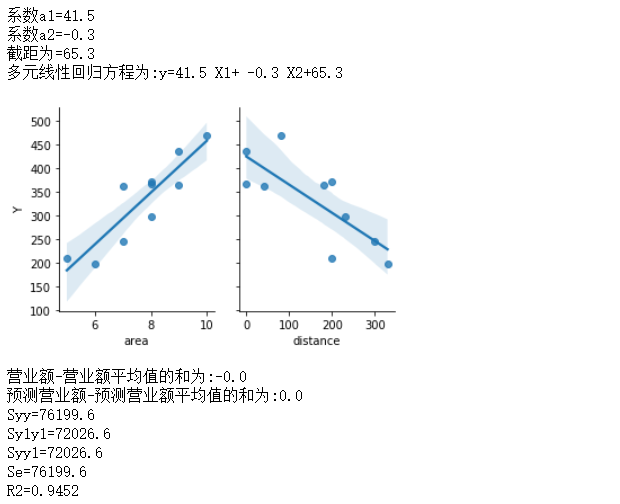2、采用sklearn库
import pandas as pd
import seaborn as sns
import matplotlib.pyplot as plt
from sklearn import linear_model
%matplotlib inline

data=pd.read_excel('D:\面积距离车站数据.xlsx')      #导入数据
X=data[['area','distance']]           #x,y取值
y=data['Y']
y=data.Y
model = linear_model.LinearRegression()
model.fit(X,y)
print("系数a1={:.1f}".format(model.coef_))
print("系数a2={:.1f}".format(model.coef_))
print("截距为={:.1f}".format(model.intercept_))
print("多元线性回归方程为:y={:.1f}".format(model.coef_),"X1+ {:.1f}".format(model.coef_),"X2+{:.1f}".format(model.intercept_))
sns.pairplot(data, x_vars=['area','distance'], y_vars='Y', height=3, aspect=0.8, kind='reg')
plt.show()

运行结果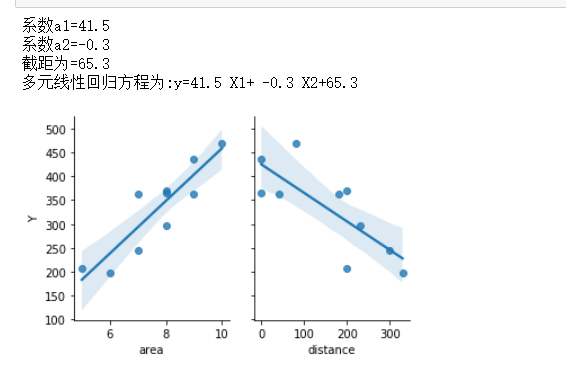（四）将梯度下降法与最小二乘法进行对比
这里给出利用Excel表格的数据分析功能得出的数据：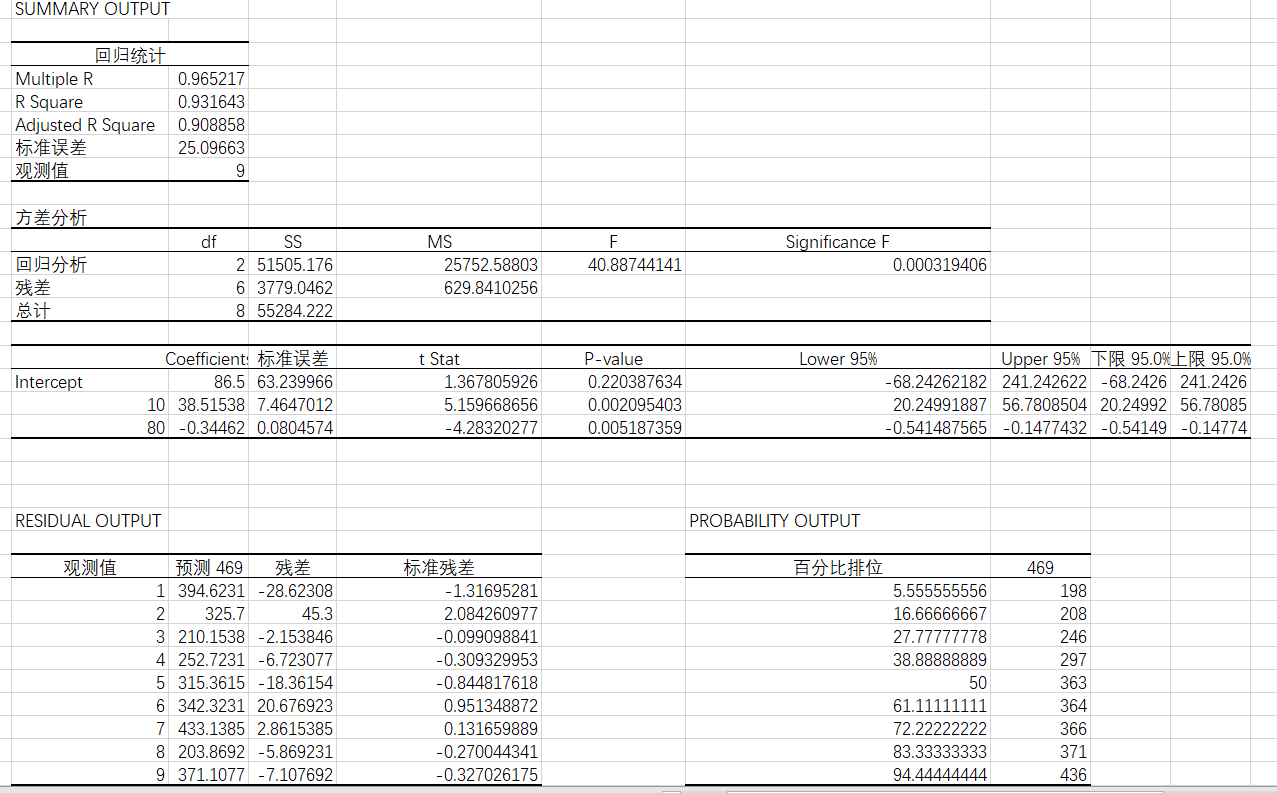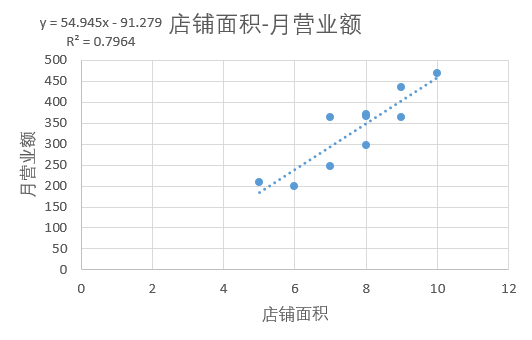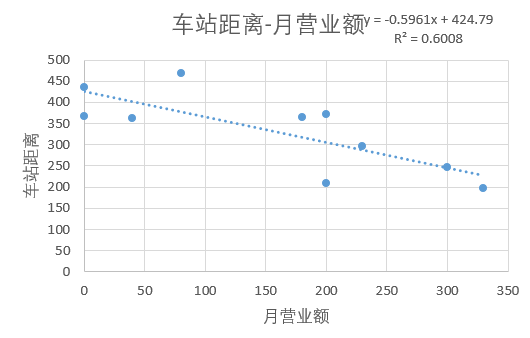通过对两种方法的对比分析，不难得出结论：最小二乘法计算量偏大，而且求逆矩阵相当耗费时间，求逆矩阵也会存在数值不稳定的情况，相比之下梯度下降法可以看做是一种更简单的最小二乘法最后一步解方程的方法，梯度下降法虽然有一定的缺点，但是计算量不大，在数据量比较大的时候选择梯度下降法比较好一点。


展开全文机器学习
• 最小二乘法系数与常数的求解公式 例子 #最小二乘法 X = [0.698132, 0.959931, 1.134464, 1.570796, 1.919862] Y = [0.188224, 0.209138, 0.230052, 0.250965, 0.313707] n = len(X) xy, x, y, x2, = 0, 0, 0, 0 ...
线性最小二乘法系数与常数的求解公式例子#线性最小二乘法
X = [0.698132, 0.959931, 1.134464, 1.570796, 1.919862]
Y = [0.188224, 0.209138, 0.230052, 0.250965, 0.313707]

n = len(X)
xy, x, y, x2,  = 0, 0, 0, 0

for i in range(n):
xy += X[i]*Y[i]  #xy的和
x += X[i]  #x的和
y += Y[i]  #y的和
x2 += X[i]**2  #x的平方的和

a1 = (n*xy - x*y) / (n*x2 - x**2)  #系数K
a0 = (x2*y - x*xy) / (n*x2 - x**2)  #常数b
print('a1 =',a1)
print('a0 =',a0)

结果：
a1 = 0.09609143373278023
a0 = 0.11766514898834005

因此线性最小二乘拟合为
T = 0.09609143373278023 * θ + 0.11766514898834005
特殊点的线性最小二乘法，即截距为0
如果曲线过（0，0）点，线性最小二乘法的拟合曲线的截距应为0，那么其斜率的计算又是另一种公式了。代码：
#线性最小二乘法
X = [0, 0.183, 0.36, 0.5324, 0.702, 0.867, 1.0244, 1.1774, 1.329, 1.479, 1.5, 1.56]
Y = [0, 306, 612, 917, 1223, 1529, 1835, 2140, 2446, 2752, 2767, 2896]

n = len(X)
xy, x, y, x2,  = 0, 0, 0, 0

if X.index(0) == Y.index(0):
a0 = 0  #常数b为0,即截距为0
for i in range(n):
xy += X[i]*Y[i]  #xy的和
x2 += X[i]**2  #x的平方的和
a1 = xy / x2  #系数K
print('a1 =',a1)
print('a0 =',a0)
else:
for i in range(n):
xy += X[i]*Y[i]  #xy的和
x += X[i]  #x的和
y += Y[i]  #y的和
x2 += X[i]**2  #x的平方的和

a1 = (n*xy - x*y) / (n*x2 - x**2)  #系数K
a0 = (x2*y - x*xy) / (n*x2 - x**2)  #常数b
print('a1 =',a1)
print('a0 =',a0)

结果：
a1 = 1828.3740666629756
a0 = 0

上面的程序只能在有（0,0）的情况下正常运行，如果还存在（0，y）和（x，0）就不能正常运行。
所以进行了改进：
#线性最小二乘法
X = [0, 0.183, 0.36, 0.5324, 0.702, 0.867, 1.0244, 1.1774, 1.329, 1.479, 1.5, 1.56]
Y = [0, 306, 612, 917, 1223, 1529, 1835, 2140, 2446, 2752, 2767, 2896]

n = len(X)
xy, x, y, x2,  = 0, 0, 0, 0
flag = 1
#判断趋势线过不过(0,0)点
for i,j in zip(X,Y):
if i ==0 and j == 0:
flag = 0
#求解系数
if flag == 0:
a0 = 0  #常数b为0,即截距为0
for i in range(n):
xy += X[i]*Y[i]  #xy的和
x2 += X[i]**2  #x的平方的和
a1 = xy / x2  #系数K
print('a1 =',a1)
print('a0 =',a0)
else:
for i in range(n):
xy += X[i]*Y[i]  #xy的和
x += X[i]  #x的和
y += Y[i]  #y的和
x2 += X[i]**2  #x的平方的和

a1 = (n*xy - x*y) / (n*x2 - x**2)  #系数K
a0 = (x2*y - x*xy) / (n*x2 - x**2)  #常数b
print('a1 =',a1)
print('a0 =',a0)

结果：
a1 = 1828.3740666629756
a0 = 0



展开全文算法
• 基于jupyter notebook的python编程—–利用梯度下降算法求解多元线性回归方程，并与最小二乘法求解进行精度对比目录一、梯度下降算法的基本原理1、梯度下降算法的基本原理二、题目、表格数据、以及python环境搭建1、...
• 在感性评价值（因变量）Y与造型设计要素各类别的反应之间存在线性关系，因此可以得到线性模型： 对于上边的多元线性回归模型的求解，我们可以用最小二乘法来求做逼近求得其近似解（系数ajk）。 求得的ajk 即为类别...
用观察数据（实验数据）来研究一些随机变量之间的关系时常用各种各样的多元分析方法。由于随机变量所处的背景的不同，我们大致可以把随机变量分为两类：一类是常规的变量（数量有变化的变量），例如重量、价格、时间、速度等；另一种变量不是真的在数量上有变化的变量，只是在性质上有变化，例如三角形变化为正方形，这种性质上发生变化的变量我们通常称为定性变量或名义尺度变量。由定性变量组成的数据为定性数据，而分析定量数据的多元分析方法，则不能解决此类问题。为了解决此类问题，在20世纪50年代产生了专门为解决各种定性研究数据的方法，这种方法就是数量化理论。数量化理论有很多，这里介绍的数量化一类理论是感性工学研究中应用最多的一种。数量化一类理论是研 究一组定性变量X（自 变量）与一组定量变量ｙ（因变量）之间的关系，将定性的变量进行量化，变成０、１等定量的数据后，利用多元线性回归分析，建立它们之间的数学模型，从而实现对因变量Y的预测。在产品造型设计的感性工学研究中，我们常常需要去研究某个产品的形态 （如：壁灯的形态）与用户的感性评价值（如：用户对壁灯A的新颖性评价值，就是用户的感性评价值）之间的关系。这就是一种典型的数量化一类理论的运用。比如我们有N个样本（N个同类的产品，比如有N个壁灯），我们对这N个样本进行造型设计要素的分解（以系统观念来看，一个产品可由不同的设计元素组合而成 ，而每个设计元素又有不同的形态，因而形成了不同造型的产品。）。将造型设计要素作为自变量 X(定性变量)，感性评价值作为因变量Y (定量变量)，设有m个造型设计要素，第 j 个造型设计要素的类别数目由Cj 表示，δi ( j , k) 称为第 j 个造型设计要素第 k 类在第 i 组样品中的反应 ,则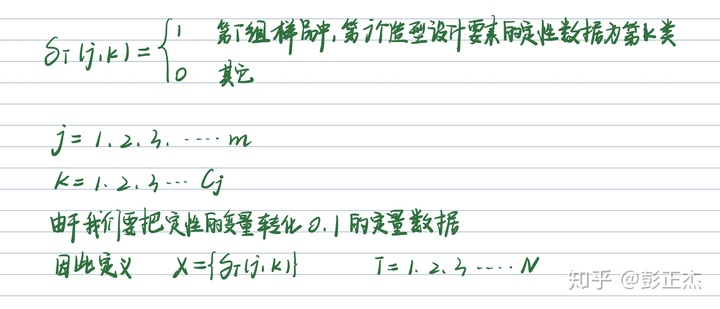在感性评价值（因变量）Y与造型设计要素各类别的反应之间存在线性关系，因此可以得到线性模型：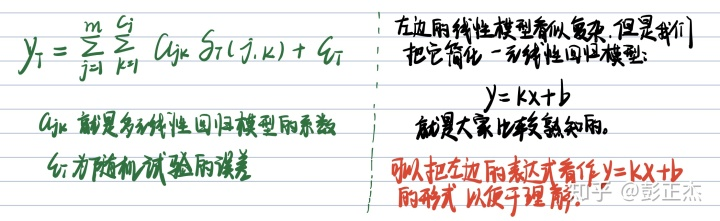对于上边的多元线性回归模型的求解，我们可以用最小二乘法来求做逼近求得其近似解（系数ajk）。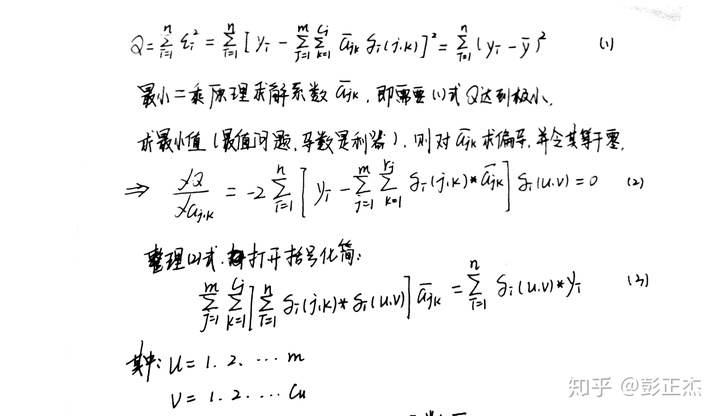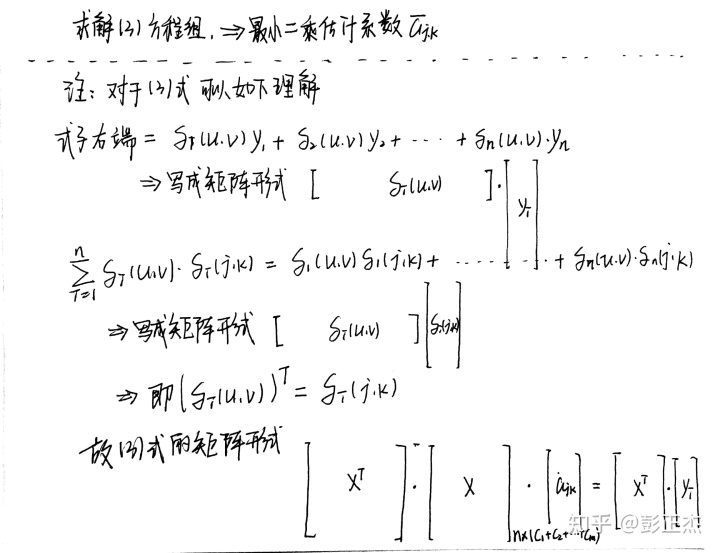求得的ajk 即为类别（每个设计要素的Cj类形态）对项目（m个造型设计要素）的贡献度，ajk越大说明其对应的类别对项目的贡献度越大。下面是Python实现程序：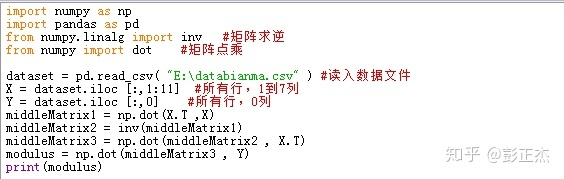Python计算系数的程序代码测试代码数据：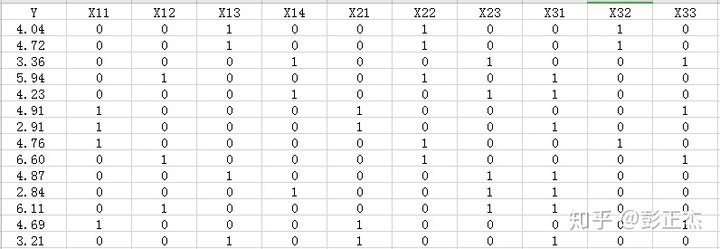程序运行结果：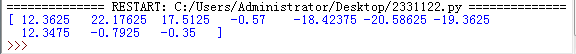展开全文• Python MATLAB MATLAB和Python数值和科学计算 数学背景 本节介绍矢量范数的概念以及矢量空间Rn\mathbb{R}^nRn中序列的收敛性。 收敛序列与Cauchi收敛 设a为R\mathbb{R}^{}R中的任意点，而ε∈R+\varepsilon \in \...
Python      MATLAB
MATLAB和Python全文档
数学背景
本节介绍矢量范数的概念以及矢量空间$\mathbb{R}^n$中序列的收敛性。
收敛序列与Cauchi收敛
设a为$\mathbb{R}^{}$中的任意点，而$\varepsilon \in \mathbb{R}^+$为任何正实数。 a的$\varepsilon$ 邻域（用$\mathscr{N}\left( \alpha ,\varepsilon \right)$ 表示）是位于开放区间$\left( \alpha -\varepsilon ,\alpha +\varepsilon \right)$ 中的所有点$\chi \in \mathbb{R}$的集合。 也就是说，$\mathscr{N}\left( \alpha ,\varepsilon \right) =\left\{ \chi \in \mathbb{R}:\left| \chi -\alpha \right|<\varepsilon \right\}$ 。
实数序列$\left\{ a_n \right\} _{n=0}^{\infty}=a_{0,}a_1,a_2,\cdots$ 表示收敛到$\alpha \in \mathbb{R}$，如果对于任何$\varepsilon >0$的选择，$\mathscr{N}\left( \alpha ,\varepsilon \right)$ 包含一个$\left\{ a_n \right\} _{n=0}^{\infty}$无限子序列$\left\{ a_n \right\} _{n=N}^{\infty}=a_{N,}a_{N+1},a_{N+2},\cdots$ 。为了数学上表达序列$\left\{ a_n \right\} _{n=0}^{\infty}$的收敛性，我们写成
$\left| a_n-a \right|<\varepsilon ,\forall _n\geqslant N$
例如，序列
$当n\rightarrow \infty 时\left\{ \frac{n}{n+1} \right\} _{n=0}^{\infty}\rightarrow 1$
因为，如果我们对$\varepsilon >0$作任何选择，那么
$\left| \frac{n}{n+1}-1 \right|=\left| \frac{-1}{n+1} \right|=\frac{1}{n+1}<\varepsilon \Longrightarrow n>\frac{1}{\varepsilon}-1=\frac{1-\varepsilon}{\varepsilon}$
因此，如果我们让
$N=\left| \frac{1-\varepsilon}{\varepsilon} \right|$
那么对于任何$n\geqslant N$的整数，我们发现
$\left| \frac{n}{n+1}-1 \right|<\varepsilon$
向量范数
如果x是具有分量$x_{1,}x_{2,}\cdots ,x_n$的向量，则其$p^{th}$-范数定义为：
$\left\| x \right\| _p=\left( \sum_{j=1}^n{\left| x_j \right|^p} \right) ^{\frac{1}{p}}=\left( \left| x_1 \right|^p+\left| x_2 \right|^p+\cdots +\left| x_n \right|^p \right) ^{\frac{1}{p}}$
满足$\left\| x \right\| _1\geqslant \left\| x \right\| _2\geqslant \cdots \geqslant \left\| x \right\| _{\infty}$。 范数$\left\| x \right\| _2$给出了经典的欧几里得距离：
$\left\| x \right\| _2=\sqrt{x_{1}^{2}+x_{2}^{2}+\cdots x_{n}^{2}}$
Python函数norm（位于numpy.linalg库中）接收向量x和整数p或np.inf，并返回$\left\| x \right\| _p$。
In : x = np.array([1, -1, 2, -2, 3, -3])
In : from numpy.linalg import norm
In : n1, n2, n3 = norm(x, 1), norm(x, 2), norm(x, np.inf)
In : print(n1, n2, n3)
12.0
5.29150262213
3.0

向量的收敛序列
向量空间中的距离可以使用任何范数来定义。 如果我们有两个向量x和y都在$\mathbb{R}^n$中，则x和y之间的距离可以由$\left\| x-y \right\| _{1,}\left\| x-y \right\| _{2,}\cdots 或\left\| x-y \right\| _{\infty}$定义。 通常，将$\left\| x-y \right\| _{2,}或\left\| x-y \right\| _{\infty}$用作两个向量x和y之间距离的值。
迭代方法
解决方程组线性系统的迭代方法是从给定的初始猜测$x^{\left( 0 \right)}$开始并生成向量$x^{\left( 0 \right)},x^{\left( 1 \right)},\cdots$ 的序列，收敛到线性系统$x=x^*$的解。 生成的序列在满足$\left\| x^{\left( s \right)}-x^{\left( s-1 \right)} \right\| <\varepsilon$的某些向量x（s）处停止。
其中$\left\| \right\|$ 是$\mathbb{R}^n$中的一些范数，并且ε> 0是给定的公差。 然后，线性系统$x^*$的解近似为$x^{\left( s \right)}$，即$x^*\approx x^{\left( s \right)}$。
通常，有两种迭代方法：

静态迭代方法：在迭代k时，迭代方法从$x^{\left( k-1 \right)}$计算$x^{\left( k \right)}$，而无需参考先前的历史记录。 这类方法包括Jacobi方法，Gauss-Seidel方法和松弛方法。
非平稳迭代方法：在迭代k时，迭代方法引用整个历史$x^{\left( 0 \right)},x^{\left( 1 \right)},\cdots ,x^{\left( k-1 \right)}$来计算$x^{\left( k \right)}$。 这类方法包括共轭梯度法和GMRES子空间法。

整体思路
给定线性系统Ax = b，ε> 0且初始点$x^{\left( 0 \right)}$。 目的是在$\mathbb{R}^n$中生成向量序列：
$x^{\left( 0 \right)},x^{\left( 1 \right)},x^{\left( 2 \right)},\cdots$
收敛到线性系统$Ax=b$的解，即是$\underset{k\rightarrow \infty}{\lim}x^{\left( k \right)}=x^*$。
序列的生成在某些迭代s处停止，如
$\left\| x^{\left( s \right)}-x^{\left( s-1 \right)} \right\| <\varepsilon$
在平稳迭代方法中，矩阵A表示为两个矩阵S和T的总和，即A = S T，其中S是可逆矩阵。 然后，将线性系统Ax = b替换为$\left( S+T \right) x=b$或$Sx=b-Tx$。
由于S是可逆的，所以我们将两侧乘以S -1并得到一个线性系统：$x=Bx+c$。
其中$B=S^{-1}T$和$C=S^{-1}b$。
上述线性系统的解是不动点$x^*$，其中$x^*=Bx^*+c$。
从给定的初始点$x^{\left( 0 \right)}$开始，通过使用迭代关系$x^{\left( k+1 \right)}=Bx^{\left( k \right)}+c$来迭代逼近固定点。
在本节的整个过程中，我们假设矩阵A是三个矩阵L，D和U的和，其中：
$L=\left( \begin{matrix}{l} 0& 0& 0& \cdots& 0& 0& 0\\ a_{2,1}& 0& 0& \cdots& 0& 0& 0\\ a_{3,1}& a_{3,2}& 0& \cdots& 0& 0& 0\\ \vdots& \vdots& \vdots& \ddots& \vdots& \vdots& \vdots\\ a_{n-2,1}& a_{n-2,2}& a_{n-2,3}& \cdots& 0& 0& 0\\ a_{n-1,1}& a_{n-1,2}& a_{n-1,3}& \cdots& a_{n-1,n-2}& 0& 0\\ a_{n,1}& a_{n,2}& a_{n,3}& \cdots& a_{n,n-2}& a_{n,n-1}& 0\\\end{matrix} \right)$
$\boldsymbol{D}=\left( \begin{matrix}{l} \boldsymbol{a}_{1,1}& 0& 0& \cdots& 0& 0& 0\\ 0& \boldsymbol{a}_{2,2}& 0& \cdots& 0& 0& 0\\ 0& 0& \boldsymbol{a}_{3,3}& \cdots& 0& 0& 0\\ \vdots& \vdots& \vdots& \ddots& \vdots& \vdots& \vdots\\ 0& 0& 0& \cdots& \boldsymbol{a}_{\boldsymbol{n}-2,\boldsymbol{n}-2}& 0& 0\\ 0& 0& 0& \cdots& 0& \boldsymbol{a}_{\boldsymbol{n}-1,\boldsymbol{n}-1}& 0\\ 0& 0& 0& \cdots& 0& 0& \boldsymbol{a}_{\boldsymbol{n},\boldsymbol{n}}\\\end{matrix} \right)$
$\boldsymbol{U}=\left( \begin{matrix}{l} 0& \boldsymbol{a}_{1,2}& \boldsymbol{a}_{1,3}& \cdots& \boldsymbol{a}_{1,\boldsymbol{n}-2}& \boldsymbol{a}_{1,\boldsymbol{n}-1}& \boldsymbol{a}_{1,\boldsymbol{n}}\\ 0& 0& \boldsymbol{a}_{2,3}& \cdots& \boldsymbol{a}_{2,\boldsymbol{n}-2}& \boldsymbol{a}_{2,\boldsymbol{n}-1}& \boldsymbol{a}_{2,\boldsymbol{n}}\\ 0& 0& 0& \cdots& \boldsymbol{a}_{3,\boldsymbol{n}-2}& \boldsymbol{a}_{3,\boldsymbol{n}-1}& \boldsymbol{a}_{3,\boldsymbol{n}}\\ \vdots& \vdots& \vdots& \ddots& \vdots& \vdots& \vdots\\ 0& 0& 0& \cdots& 0& \boldsymbol{a}_{\boldsymbol{n}-2,\boldsymbol{n}-1}& \boldsymbol{a}_{\boldsymbol{n}-2,\boldsymbol{n}}\\ 0& 0& 0& \cdots& 0& 0& \boldsymbol{a}_{\boldsymbol{n}-1,\boldsymbol{n}}\\ 0& 0& 0& \cdots& 0& 0& \boldsymbol{a}_{\boldsymbol{n},\boldsymbol{n}}\\\end{matrix} \right)$
在MATLAB中，可以使用以下命令获得矩阵L，D和U：
>> D = diag(diag(A)) ;
>> L = tril(A) - D ;
>> U = triu(A) - D;

在Python中，tril，triu在scipy.linalg包中实现，而diag在scipy和numpy中都实现。 可以通过以下命令获得它们：
In : import scipy as sp, numpy as np
In : A = np.array([[4, 1, -1], [-1, 5, 1], [0, -1, 4]])
In : print(’L = \n’, sp.linalg.tril(A), ’\nU = \n’,
sp.linalg.triu(A), ...
’\nD = \n’, np.diag(np.diag(A)))
L =
[[ 4 0 0]
[-1 5 0]
[ 0 -1 4]]
U =
[[ 4 1 -1]
[ 0 5 1]
[ 0 0 4]]
D =
[[4 0 0]
[0 5 0]
[0 0 4]]

雅可比法
给定方程的线性系统：
$\boldsymbol{a}_{11}\boldsymbol{x}_1+\boldsymbol{a}_{12}\boldsymbol{x}_2+\cdots +\boldsymbol{a}_{1\boldsymbol{n}}\boldsymbol{x}_{\boldsymbol{n}}=\boldsymbol{b}_1\\\boldsymbol{a}_{21}\boldsymbol{x}_1+\boldsymbol{a}_{22}\boldsymbol{x}_2+\cdots +\boldsymbol{a}_{2\boldsymbol{n}}\boldsymbol{x}_{\boldsymbol{n}}=\boldsymbol{b}_2\\\vdots \\\boldsymbol{a}_{\boldsymbol{i}1}\boldsymbol{x}_1+\boldsymbol{a}_{\boldsymbol{i}2}\boldsymbol{x}_2+\cdots +\boldsymbol{a}_{\boldsymbol{in}}\boldsymbol{x}_{\boldsymbol{n}}=\boldsymbol{b}_{\boldsymbol{i}}\\\vdots \\\boldsymbol{a}_{\boldsymbol{n}1}\boldsymbol{x}_1+\boldsymbol{a}_{\boldsymbol{n}2}\boldsymbol{x}_2+\cdots +\boldsymbol{a}_{\boldsymbol{nn}}\boldsymbol{x}_{\boldsymbol{n}}=\boldsymbol{b}_{\boldsymbol{b}}$
从上面的等式可以得出：
$\boldsymbol{x}_1=\frac{1}{\boldsymbol{a}_{11}}\left( \boldsymbol{b}_1-\boldsymbol{a}_{12}\boldsymbol{x}_2-\cdots -\boldsymbol{a}_{\boldsymbol{n}1}\boldsymbol{x}_{\boldsymbol{n}} \right) \\\boldsymbol{x}_2=\frac{1}{\boldsymbol{a}_{22}}\left( \boldsymbol{b}_2-\boldsymbol{a}_{21}\boldsymbol{x}_1-\boldsymbol{a}_{23}\boldsymbol{x}_3-\cdots -\boldsymbol{a}_{\boldsymbol{n}1}\boldsymbol{x}_{\boldsymbol{n}} \right) \\\vdots \,\, \vdots \\\boldsymbol{x}_{\boldsymbol{i}}=\frac{1}{\boldsymbol{a}_{\boldsymbol{ii}}}\left( \boldsymbol{b}_{\boldsymbol{i}}-\boldsymbol{a}_{\boldsymbol{i}1}\boldsymbol{x}_1-\cdots -\boldsymbol{a}_{\boldsymbol{ii}-1}\boldsymbol{x}_{\boldsymbol{i}-1}-\boldsymbol{a}_{\boldsymbol{ii}+1}\boldsymbol{x}_{\boldsymbol{i}+1}-\cdots -\boldsymbol{a}_{\boldsymbol{in}}\boldsymbol{x}_{\boldsymbol{n}} \right) \\\vdots \,\, \vdots \\\boldsymbol{x}_{\boldsymbol{n}}=\frac{1}{\boldsymbol{a}_{\boldsymbol{nn}}}\left( \boldsymbol{b}_{\boldsymbol{n}}-\boldsymbol{a}_{\boldsymbol{n}1}\boldsymbol{x}_1-\cdots -\boldsymbol{a}_{\boldsymbol{nn}-1}\boldsymbol{x}_{\boldsymbol{n}-1} \right)$
雅可比法是一种迭代方法，它从对解$\left[ \boldsymbol{x}_{1}^{\left( 0 \right)},\boldsymbol{x}_{2}^{\left( 0 \right)},\cdots ,\boldsymbol{x}_{\boldsymbol{n}}^{\left( 0 \right)} \right] ^{\boldsymbol{T}}$的初始猜测开始。 然后，使用迭代$k$中的解来找到迭代$k+1$中系统解的近似值。
完成如下：
$\boldsymbol{x}_{1}^{\left( \boldsymbol{k}+1 \right)}=\frac{1}{\boldsymbol{a}_{11}}\left( \boldsymbol{b}_1-\boldsymbol{a}_{12}\boldsymbol{x}_{2}^{\left( \boldsymbol{k} \right)}-\cdots -\boldsymbol{a}_{\boldsymbol{n}1}\boldsymbol{x}_{\boldsymbol{n}}^{\left( \boldsymbol{k} \right)} \right) \\\boldsymbol{x}_{2}^{\left( \boldsymbol{k}+1 \right)}=\frac{1}{\boldsymbol{a}_{22}}\left( \boldsymbol{b}_2-\boldsymbol{a}_{21}\boldsymbol{x}_{1}^{\left( \boldsymbol{k} \right)}-\boldsymbol{a}_{23}\boldsymbol{x}_{3}^{\left( \boldsymbol{k} \right)}-\cdots -\boldsymbol{a}_{\boldsymbol{n}1}\boldsymbol{x}_{\boldsymbol{n}}^{\left( \boldsymbol{k} \right)} \right) \\\vdots \,\, \vdots \\\boldsymbol{x}_{\boldsymbol{i}}^{\left( \boldsymbol{k}+1 \right)}=\frac{1}{\boldsymbol{a}_{\boldsymbol{ii}}}\left( \boldsymbol{b}_{\boldsymbol{i}}-\boldsymbol{a}_{\boldsymbol{i}1}\boldsymbol{x}_{1}^{\left( \boldsymbol{k} \right)}-\cdots -\boldsymbol{a}_{\boldsymbol{ii}-1}\boldsymbol{x}_{\boldsymbol{i}-1}^{\left( \boldsymbol{k} \right)}-\boldsymbol{a}_{\boldsymbol{ii}+1}\boldsymbol{x}_{\boldsymbol{i}+1}^{\left( \boldsymbol{k} \right)}-\cdots -\boldsymbol{a}_{\boldsymbol{in}}\boldsymbol{x}_{\boldsymbol{n}}^{\left( \boldsymbol{k} \right)} \right) \\\vdots \,\, \vdots \\\boldsymbol{x}_{\boldsymbol{n}}^{\left( \boldsymbol{k}+1 \right)}=\frac{1}{\boldsymbol{a}_{\boldsymbol{nn}}}\left( \boldsymbol{b}_{\boldsymbol{n}}-\boldsymbol{a}_{\boldsymbol{n}1}\boldsymbol{x}_{1}^{\left( \boldsymbol{k} \right)}-\cdots -\boldsymbol{a}_{\boldsymbol{nn}-1}\boldsymbol{x}_{\boldsymbol{n}-1}^{\left( \boldsymbol{k} \right)} \right)$
通常，迭代$\boldsymbol{x}_{\boldsymbol{i}}^{\left( \boldsymbol{k}+1 \right)}$中的解可以表示为：
$\boldsymbol{x}_{\boldsymbol{i}}^{\left( \boldsymbol{k}+1 \right)}=\frac{1}{\boldsymbol{a}_{\boldsymbol{ii}}}\left( \boldsymbol{b}_{\boldsymbol{i}}-\sum_{\boldsymbol{j}=1,\boldsymbol{j}\ne \boldsymbol{i}}^{\boldsymbol{n}}{\boldsymbol{a}_{\boldsymbol{ij}}\boldsymbol{x}_{\boldsymbol{j}}^{\left( \boldsymbol{k} \right)}} \right) ,\boldsymbol{i}=1,\cdots ,\boldsymbol{n}$
对于任意$\boldsymbol{\varepsilon }>0$，当$\left\| \boldsymbol{x}_{\boldsymbol{i}}^{\left( \boldsymbol{k}+1 \right)}-\boldsymbol{x}_{\boldsymbol{i}}^{\left( \boldsymbol{k} \right)} \right\| <\boldsymbol{\varepsilon }$时，雅可比迭代停止。
**示例1，**为线性系统编写雅可比方法的前三个迭代：
$\left( \begin{matrix} 2& -1& 1\\ -2& 5& -1\\ 1& -2& 4\\\end{matrix} \right) \left( \begin{array}{c} \boldsymbol{x}_1\\ \boldsymbol{x}_2\\ \boldsymbol{x}_3\\\end{array} \right) =\left( \begin{array}{c} -1\\ 1\\ 3\\\end{array} \right)$
从零向量开始
$\boldsymbol{x}^{\left( 0 \right)}=\left( \begin{array}{c} 0\\ 0\\ 0\\\end{array} \right)$
解决
我们写成：
$\boldsymbol{x}_{1}^{\left( \boldsymbol{k}+1 \right)}=\frac{1}{2}\left( -1+\boldsymbol{x}_{2}^{\left( \boldsymbol{k} \right)}-\boldsymbol{x}_{3}^{\left( \boldsymbol{k} \right)} \right) \\\boldsymbol{x}_{2}^{\left( \boldsymbol{k}+1 \right)}=\frac{1}{5}\left( 1+2\boldsymbol{x}_{1}^{\left( \boldsymbol{k} \right)}+\boldsymbol{x}_{3}^{\left( \boldsymbol{k} \right)} \right) \\\boldsymbol{x}_{3}^{\left( \boldsymbol{k}+1 \right)}=\frac{1}{4}\left( 3-\boldsymbol{x}_{1}^{\left( \boldsymbol{k} \right)}+2\boldsymbol{x}_{2}^{\left( \boldsymbol{k} \right)} \right)$

第一次迭代$k=0$
$\boldsymbol{x}_{1}^{\left( 1 \right)}=\frac{1}{2}\left( -1+\boldsymbol{x}_{2}^{\left( 0 \right)}-\boldsymbol{x}_{3}^{\left( 0 \right)} \right) =\frac{1}{2}\left( -1+0-0 \right) =-\frac{1}{2}\\\boldsymbol{x}_{2}^{\left( 1 \right)}=\frac{1}{5}\left( 1+2\boldsymbol{x}_{1}^{\left( 0 \right)}+\boldsymbol{x}_{3}^{\left( 0 \right)} \right) =\frac{1}{5}\left( 1+2\left( 0 \right) +0 \right) =\frac{1}{5}\\\boldsymbol{x}_{3}^{\left( 1 \right)}=\frac{1}{4}\left( 3-\boldsymbol{x}_{1}^{\left( 0 \right)}+2\boldsymbol{x}_{2}^{\left( 0 \right)} \right) =\frac{1}{4}\left( 3-0+2\left( 0 \right) \right) =\frac{3}{4}$

第二次迭代$k=1$
$\boldsymbol{x}_{1}^{\left( 2 \right)}=\frac{1}{2}\left( -1+\boldsymbol{x}_{2}^{\left( 1 \right)}-\boldsymbol{x}_{3}^{\left( 1 \right)} \right) =\frac{1}{2}\left( -1+\frac{1}{5}-\frac{3}{4} \right) =-\frac{31}{40}\\\boldsymbol{x}_{2}^{\left( 2 \right)}=\frac{1}{5}\left( 1+2\boldsymbol{x}_{1}^{\left( 1 \right)}+\boldsymbol{x}_{3}^{\left( 1 \right)} \right) =\frac{1}{5}\left( 1+2\cdot \frac{-1}{2}+\frac{3}{4} \right) =\frac{3}{20}\\\boldsymbol{x}_{3}^{\left( 2 \right)}=\frac{1}{4}\left( 3-\boldsymbol{x}_{1}^{\left( 1 \right)}+2\boldsymbol{x}_{2}^{\left( 1 \right)} \right) =\frac{1}{4}\left( 3-\frac{-1}{2}+2\frac{1}{5} \right) =\frac{39}{40}$

第三次迭代$k=2$
$\boldsymbol{x}_{1}^{\left( 3 \right)}=\frac{1}{2}\left( -1+\boldsymbol{x}_{2}^{\left( 2 \right)}-\boldsymbol{x}_{3}^{\left( 2 \right)} \right) =\frac{1}{2}\left( -1+\frac{3}{20}-\frac{39}{40} \right) =-\frac{73}{80}\\\boldsymbol{x}_{2}^{\left( 3 \right)}=\frac{1}{5}\left( 1+2\boldsymbol{x}_{1}^{\left( 2 \right)}+\boldsymbol{x}_{3}^{\left( 2 \right)} \right) =\frac{1}{5}\left( 1+2\cdot \frac{-31}{40}-\frac{-73}{80} \right) =\frac{17}{200}\\\boldsymbol{x}_{3}^{\left( 3 \right)}=\frac{1}{4}\left( 3-\boldsymbol{x}_{1}^{\left( 2 \right)}+2\boldsymbol{x}_{2}^{\left( 2 \right)} \right) =\frac{1}{4}\left( 3-\frac{31}{40}+2\frac{3}{20} \right) =\frac{163}{160}$

**示例2，**雅可比方法将用于求解线性系统：
$\left( \begin{matrix} -5& 1& -2\\ 1& 6& 3\\ 2& -1& -4\\\end{matrix} \right) \left( \begin{array}{c} \boldsymbol{x}_1\\ \boldsymbol{x}_2\\ \boldsymbol{x}_3\\\end{array} \right) =\left( \begin{array}{c} 13\\ 1\\ -1\\\end{array} \right)$
MATLAB代码
function x = JacobiSolve(A, b, Eps)
n = length(b) ;
x0 = zeros(3, 1) ;
x = ones(size(x0)) ;
while norm(x-x0, inf) ≥ Eps
x0 = x ;
for i = 1 : n
x(i) = b(i) ;
for j = 1 : n
if j , i
x(i) = x(i) - A(i, j)*x0(j) ;
end
end
x(i) = x(i) / A(i, i) ;
end

>> A = [-5 1 -2; 1 6 3; 2 -1 -4] ;
>> b = [13; 1; -1] ;
>> JacobiSolveLinSystem(A, b)
-2.0000
1.0000
-1.0000

使用Python，功能JacobiSolve具有以下代码：
def JacobiSolve(A, b, Eps):
import numpy as np
n = len(b)
x0, x = np.zeros((n, 1), 'float'), np.ones((n, 1), 'float')
while np.linalg.norm(x-x0, np.inf) ≥ Eps:
x0 = x.copy()
for i in range(n):
x[i] = b[i]
for j in range(n):
if j != i:
x[i] -= A[i][j]*x0[j]
x[i] /= A[i][i]
return x

通过调用函数JacobiSolve求解给定的线性系统，我们获得：
In : A = np.array([[-5, 1, -2], [1, 6, 3], [2, -1, -4]])
In : b = np.array([, , [-1]])
In : x = JacobiSolve(A, b, Eps)
In : print(’x = \n’, x)
Out:
x =
[[-2. ],
[ 1.00000002],
[-1.00000002]]

矩阵形式的雅可比法
向量形式的高斯-塞德尔方法
松弛法
最小二乘解
最小二乘解的一些应用
详情请参考http://viadean.com/matlab_python_interation.html#_np=169_856


展开全文matlab
• 最小二乘法有什么用？一般用它做什么事？ 我们最早接触最小二乘法是在高中的时候学的...这就得用最小二乘法求解。 总结：最小二乘法拟合数据的步骤有两步。1.首先，假设这些数据符合某种函数。而这种函数往往有几...教程 机器学习
• 这里写目录标题利用梯度下降算法求解多元线性回归方程，并与最小二乘法求解进行精度对比基于jupyter notebook的python编程**1. 导入库、数据、并为变量赋值****2.定义系数初始值以及学习率和迭代次数****3.定义最小...机器学习
• 目录1、线性回归的原理基础定义公式推导简单理解2、最小二乘法PYTHON实现0. 导入相关库1. 导入数据2. 定义损失函数3. 定义算法拟合函数4. 测试定义的函数5. 画出拟合曲线3、最小二乘简单例子 1、线性回归的原理 基础...机器学习 数据挖掘
• 最近学习李航《统计学习方法》，在github上找到了这本书对应的源码，决定自己跟着敲一...但最小二乘法的实现直接使用了scipy库中的leastsq，于是自己网上找了关于最小二乘法的矩阵求解，据此进行了简单的代码实现。...
• 在感性评价值(因变量)Y与造型设计要素各类别的反应之间存在线性关系，因此可以得到线性模型： 对于上边的多元线性回归模型的求解，我们可以用最小二乘法来求做逼近求得其近似解(系数ajk)。 求得的ajk 即为类别(每个...
• 最小二乘法是机器学习求解最优化问题的最常见的方法之一，掌握并熟知是对求解最优解问题更深刻的认知。
• 依旧使用最小二乘法求解Ax=b——————1无解下的最优解。已知点的个数为n，所求直线的方程为y1=ax1+b，A由方程右边的a，b的系数构成构成(nx2)的矩阵,每行为(x1,1)，b由已知点的y1坐标构成矩阵(nx...
• 其实最小二乘法为分类回归算法的基础，从求解线性透视图中的消失点，m元n次函数的拟合，包括后来学到的神经网络，其思想归根结底全都是最小二乘法。本文向大家介绍python中的最小二乘法。一、最小二乘法是什么最小...
• 依旧使用最小二乘法求解Ax=b——————1无解下的最优解。已知点的个数为n，所求直线的方程为y1=ax1+b，A由方程右边的a，b的系数构成构成(nx2)的矩阵,每行为(x1,1)，b由已知点的y1坐标构成矩阵(nx...
• 梯度下降算法是迭代法的一种,可以用于求解最小二乘问题(线性和非线性都可以)，在求解机器学习算法的模型参数，即无约束优化问题时，梯度下降（Gradient Descent）是最常采用的方法之一。 在机器学习中，基于基本的...人工智能 机器学习 梯度下降算法
• 最小二乘法非常简单，我把它分成两种视角描述： （1）已知多条近似交汇于同一个点的直线，想求解出一个近似交点：寻找到一个距离所有直线距离平方和最小的点，该点即最小二乘解； （2）已知多个近似分布于同一直线...
• 2、最小二乘法PYTHON实现 0. 导入相关库 import numpy as np import matplotlib.pyplot as plt 1. 导入数据 points = np.genfromtxt('data.csv', delimiter=',') #生成points数组 points[0,0] # 提取points中的两列......

# 最小二乘法求解pythonpython 订阅